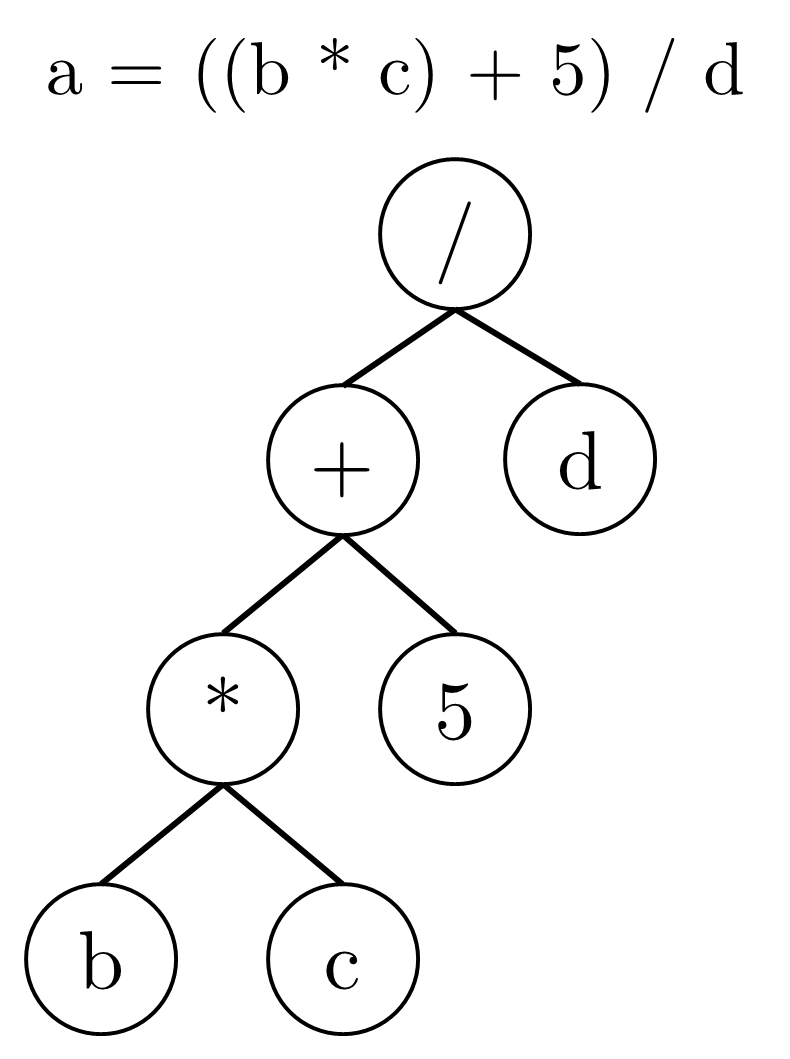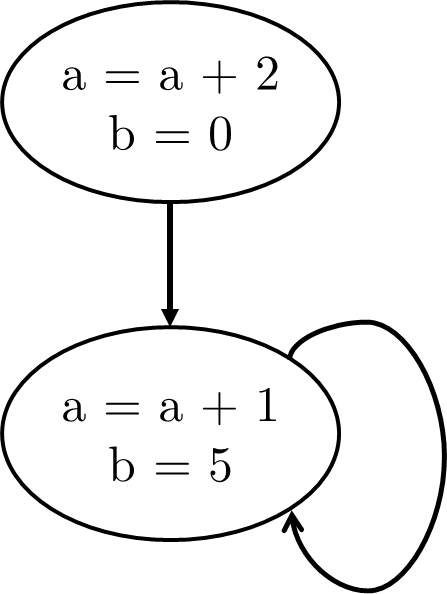# Static Single Assignments (January Haskell Tests 2018 – Part 2)Second in a two-part series; see Constant Propagation (January Haskell Tests 2018 Part 1) for the first part, which covered the assessed portion of the test.

In January this year, Imperial College’s first-year computing students were tasked with implementing a tool to handle constant propagation for statements in static single assignment (SSA) form. In particular, this means that each variable is only assigned to once, and there are no compound expressions. This is useful for optimising compilers to simplify the machine code that a program compiles down to.

Of course, being required to write everything in SSA form is painful; as much as I like immutable objects, mandating their use can be costly (consider a large set, for instance, that periodically receives updates). Banning compound statements can also be troublesome; hence, SSA compilation is typically automated.

The test concluded with a challenge tasking students to implement support for converting a more general program into SSA form. The students had been working with a simple imperative language with statements to assign values to integer variables (possibly doing mathematical operations), conditional statements (if-then-else) and do-while loops.

This problem naturally decomposes into three sub-tasks: supporting SSA translation for basic blocks, conditionals and then loops. The first problem can also readily be split into two sub-problems: ensuring variables are immutable and then handling compound expressions.

#### Basic Blocks

##### Immutable Variables

I opted to begin with immutability here, as this would allow for easy testing. This is done by creating multiple versions of a variable. For example,

a = 10;
b = a + 2;
b = a + b + 8;
c = a + b;
a = c + 3;

could become

a1 = 10;
b1 = a1 + 2;
b2 = a1 + b1 + 8;
c1 = a1 + b2;
a2 = c1 + 3;

Essentially, one could implement this by keeping track of the latest version of each variable used, and incrementing the version whenever processing an assignment. Whenever handling the right-hand side of an assignment, one needs to be able to know how to reference the latest version of a variable.

-- Usage Map for handling new instances of variables
type UsageMap = [(Id, Int)]

-- Retrieves a fresh identifier for the given raw variable.
getIdentifier :: Id -> UsageMap -> (Id, Int, UsageMap)
getIdentifier "$return" map = ("$return", 0, map)
getIdentifier id []
= (id ++ "0", 0, [(id, 1)])
getIdentifier id (binding:bindings)
| id == bindingId
= (id ++ show(bindingCount), bindingCount, (id, bindingCount + 1):bindings)
| otherwise
= (returnedId, returnedCount, binding:returnedBindings)
where
(bindingId, bindingCount) = binding
(returnedId, returnedCount, returnedBindings) = getIdentifier id bindings

I’m pretty sure I wouldn’t have bothered to write a specific UsageMap type when I was still in university, though working in industry seems to have made me think more carefully about typing.

##### Compound Statements

The version of SSA in the paper also doesn’t allow compound statements (e.g. a = ((b * c) + 5) / d). However, this could be handled reasonably as arithmetic expressions can be represented in tree form.We can create temporary variables for each non-leaf node of the expression tree. For example, we define k1 = b * c, k2 = k1 + 5 and k3 = k2 / d. Some care is needed in cases where the tree is a bit more balanced (we need to know that the first argument of our plus above is k1).

Also, of course, k is only used for illustration above, as real functions could have a value actually called k! It makes sense to have a counter separate from the rest of the UsageMap, though I relied on some limits on the variable names that were mentioned in the specification. I’m not sure if I would have worked this out in first year!

#### Conditional Expressions

One needs to consider which versions of a variable are in scope. Let’s consider the following block:

a = 7
b = a * 3
if (b > 20) {
a = b + 3
b = 0
} else {
a = b - 3
b = 1
}
return a + b

After reaching the end of the if block, we can’t just use the UsageMap we have obtained from that to pass into the else block (since on an execution where we’re going in to the else block, the if block must never have run). Similarly, after reaching the end of else we can’t just use the UsageMap from that (we don’t know if the else block has run).

It is probably easier to visualise this block of code as a tree. Each node represents a possible linear execution path that we may run.The versions of variables each of the blocks read upon branching should be the version before we entered that block. Thus, we should reference the state of the UsageMap from before we entered the if block when entering the else block. We actually want something like this.When returning, we somehow need to pick one of the final values for variables that were possibly modified. This is difficult to express, but we can approximate it with a phi function, where$\phi(a, b)$ nondeterministically returns either a or b. We can thus simply pick$a4 = \phi(a2, a3)$ and$b4 = \phi(b2, b3)$, and add these statements. Finally, we would want to return$a4 + b4$. Some care needs to be taken with the implementation (for example, one must handle the case where a variable is only possibly modified correctly). This should, for the above block of code, yield:

a1 = 7
b1 = a1 * 3
if (b1 > 20) {
a2 = b1 + 3
b2 = 0
} else {
a3 = b1 - 3
b3 = 1
}
a4 = PHI(a2, a3)
b4 = PHI(b2, b3)
return a4 + b4


During the implementation I was running out of time, which unfortunately led to this monster of a helper function. It could probably be cleaned up by perhaps making a type for ‘return values of a traversal’. It’s also probably reasonable to do some kind of higher-order function on the mod-set of variables (which I computed elsewhere).

-- Compute values to be used for PHI for one variable at a time
plantIfPhi :: UsageMap -> UsageMap -> UsageMap -> UsageMap -> [Id] -> (Block, UsageMap)
plantIfPhi original afterIf afterElse final []
= ([], final)
plantIfPhi original afterIf afterElse accumulator (id:ids)
= ([Assign newId (Phi (Var varAfterIf) (Var varAfterElse))] ++ otherPhis,
final)
where
oldVersion = lookupWithFailure id original
versionAfterIf = lookupWithFailure id afterIf
versionAfterElse = lookupWithFailure id afterElse
realVersionAfterElse
= if versionAfterIf == versionAfterElse
then oldVersion
else versionAfterElse
varAfterIf = id ++ show(versionAfterIf)
varAfterElse = id ++ show(realVersionAfterElse)
(newId, _, newMap) = getIdentifier id accumulator
(otherPhis, final)
= plantIfPhi original afterIf afterElse newMap ids

#### Do-While Loops

Finally we also need to support loops. We could use a call graph structure, similar to what we had for the if-then-else conditional, except that there is a cycle.

a = a + 2
b = 0
do {
a = a + 1
b = 5
} while (a < 10)

At first glance, this looks tricky. We don’t know the number of times the loop will execute, though we do know it’s at least once.Which value of a are we reading in the loop body? Well, this is either the value from the initial set-up, or the value the previous loop left at its end. We could thus try to introduce phi-functions:

a2 = a1 + 2
b1 = 0
do {
a3 = PHI(a2, a4)
b2 = PHI(b1, b3)
a4 = a3 + 1
b3 = 5
} while (a4 < 10)

This program is impossible to execute, of course; the definitions are cyclic. However, we’re not actually trying to execute it. We’re only using this as an intermediate representation to try to statically optimise our code. Of course, if the two arguments to a phi-function are the same, we can eliminate it, mark that value as a constant if known, and then use the information about it being a constant to drive further optimisations.

#### Synthesis

I think this could be a fairly challenging question in and of itself for first-year computing students, though some degree of hand-holding would likely be required (in particular, the tree traversal for splitting out intermediate assignments can be quite fiddly to work with). It would also be worth introducing the concept of basic blocks early on, as reasoning about them is more useful in handling conditions and loops. It was a rather solid and satisfying conclusion to the original 2017 test.